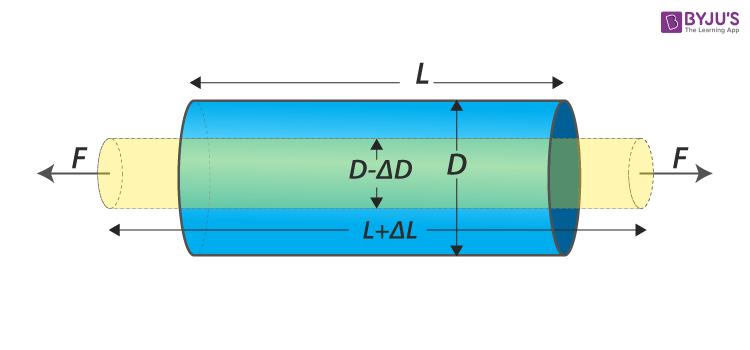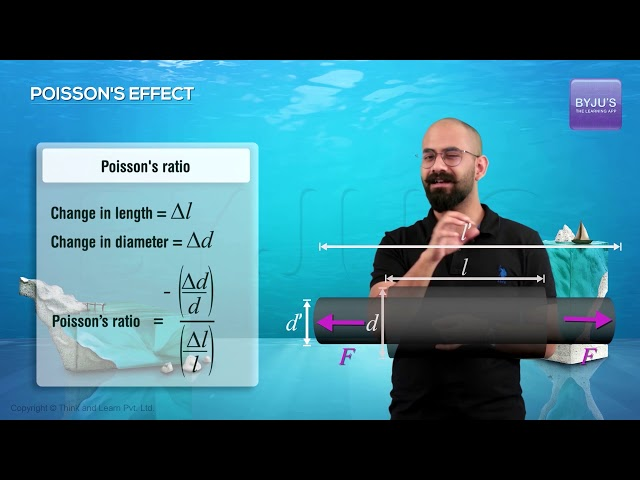# Longitudinal Strain

### There are two types of strain :

• Longitudinal strain
• Lateral strain

## What is a Longitudinal Strain?

A longitudinal strain is defined as

Change in the length to the original length of an object

It is caused due to longitudinal stress and is denoted by the Greek letter epsilon 𝜺.## Longitudinal Strain Formula

Consider a cylinder. When that longitudinal stress acts on it, there will be a change in the length of the cylinder. Then the longitudinal strain can be mathematically expressed as follows:

 $$Longitudinal Strain=\frac{Change\;in\;the\;length}{Original\;Length}$$ $$\varepsilon =\frac{\Delta L}{L}$$

Where,

• L is the original length
• ΔL change in length

### Longitudinal Strain Unit

It is expressed as $$\varepsilon =\frac{\Delta L}{L}$$. Here the fundamental unit of length is the meter. Substituting it in the formula we get:

$$SI\;Unit\;of\;Longitudinal\;Stress=\frac{m}{m}$$

They cancel each other, making it unit less or dimensionless quantity.## Longitudinal Strain Meaning

Stress and strain are like two sides of a coin. It is important to know their behaviour at microscopic scales. We know that all solids are elastic by nature. Atoms or molecules constitute them. Each atom or molecule is surrounded by neighbouring atoms or molecules. Each atom or molecule surrounded by neighbouring atoms or molecules are bonded together by intermolecular forces and stay in equilibrium. When a deforming force or external force is applied, their intermolecular or inter-atomic distances vary. As a result, a restoring force will be generated to attain back the equilibrium, thereby regaining original size and shape.

When an object is subjected to deforming force, the restoring force will be generated to oppose the deforming force and get back the original shape and size. The restoring force has magnitude same as that of applied force but in the opposite direction. This restoring force per unit area is called stress.

Consider a cylinder (figure above) with L as length and D as the diameter. What happens to the cylinder when an equal force is applied such that it is perpendicular to the cross-sectional area? The answer is there will be a change in its length as well as in diameter. The length will increase from L to L+ΔL and Diameter will reduce from D to D-ΔD. Thus, the change in dimension to the original dimension is defined as strain. It tells the amount of stress an object is experiencing.

When the object is stretched, the restoring force is known as tensile stress. When the object is compressed, the restoring force is known as compressive stress. In both cases, there will be a considerable change in the length of the object, say, cylinder. Thus we can define “longitudinal strain as Change in the Length ΔL to Original Length L”.